### SigmaPlot-Beispiele

Graph-Beispiele (1)   Graph-Beispiele (2)   Transform-Beispiele

#### 1. Graph-Beispiele: Samples.jnb

Die Datei Samples.jnb enthält in über 30 Sections Beispiele von SigmaPlot-Graphen. Sie finden die Datei im Help-Menü > "SigmaPlot Sample Graphs".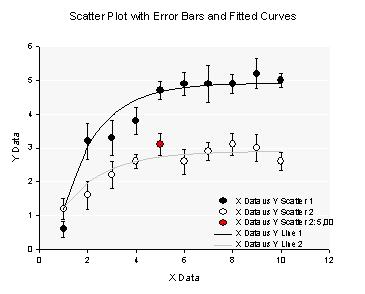Example 1:
Scatter Plot with Error Bars and Fitted Curves

Graph with 2 plots
Plot 1: Multiple Error Bars, XY Pairs
Plot 2: Multiple Spline Curves, X Many Y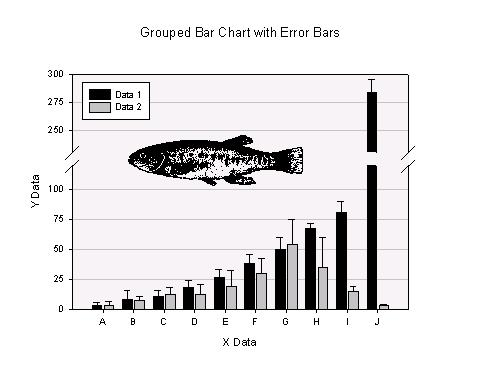Example 2:
Grouped Bar Chart with Error Bars

Plot type: Grouped Error Bars
Data format: X, Many Y Replicates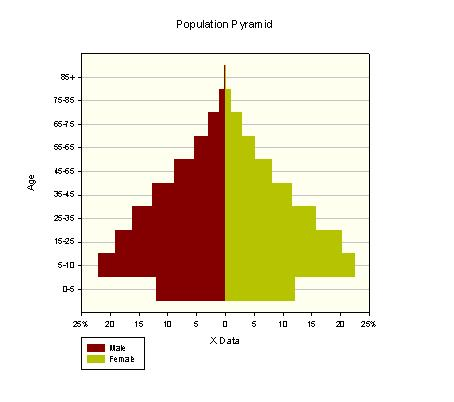Example 3:
Population Pyramid

Plot type: Stacked Bars
Data format: Y Many X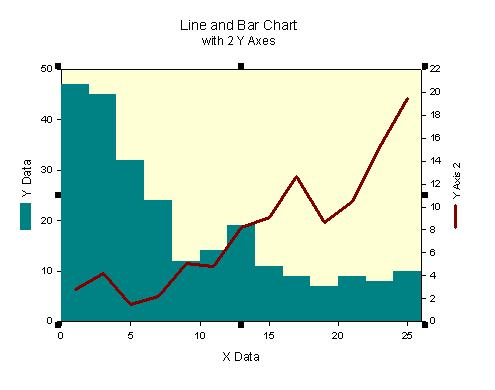Example 4:
Bar and Line Chart with Two Y Axes

Plot 1: Bar Chart, Simple Bar, XY Pair
Plot 2: Line Plot, Simple Straight Line, XY Pair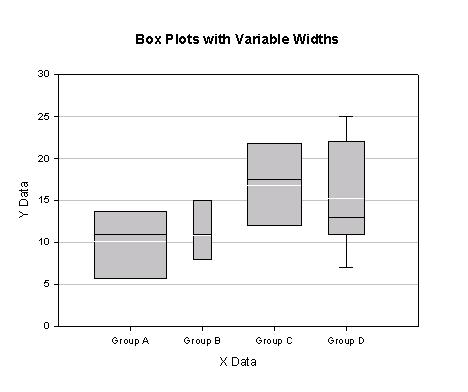Example 5:
Box Plots with Variable Widths

Plot type: Vertical Box Plot
Data format: X Many Y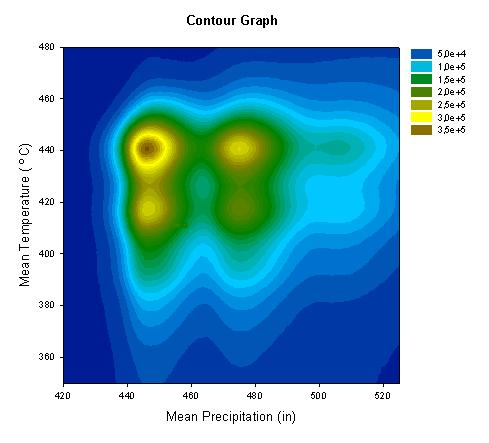Example 6:
Color Contour Graph

Plot type: Filled Contour Plot
Data format: XY Many Z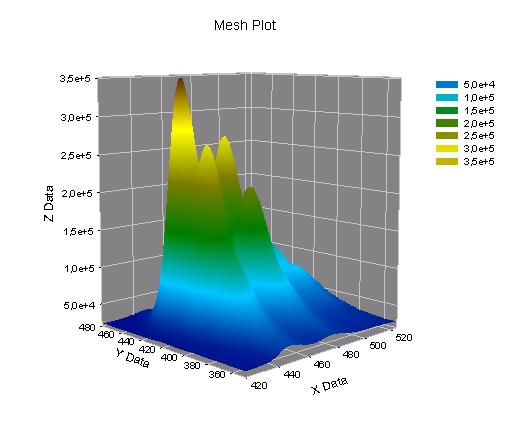Example 6:
Mesh Plot

Plot type: 3D Mesh Plot
Data format: XY Many Z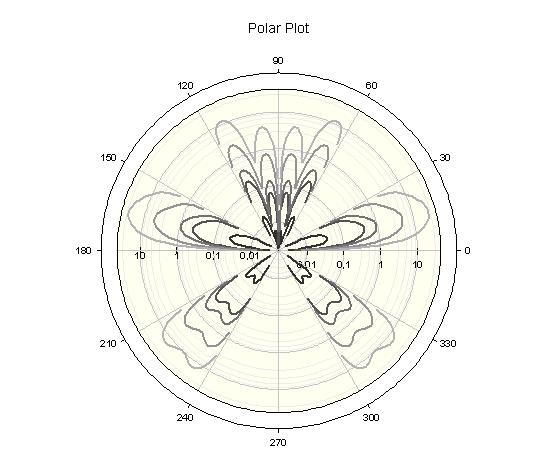Example 7:
Polar Plot

Plot type: Polar Plot, Lines
Data format: Theta Many RExample 8:
Quartile Plot

Plot 1: Multiple Error Bars, X Many Y (Black symbols and error bar lines)
Plot 2: Multiple Error Bars, X Many Y (Dk. Red symbols and error bar "gaps":)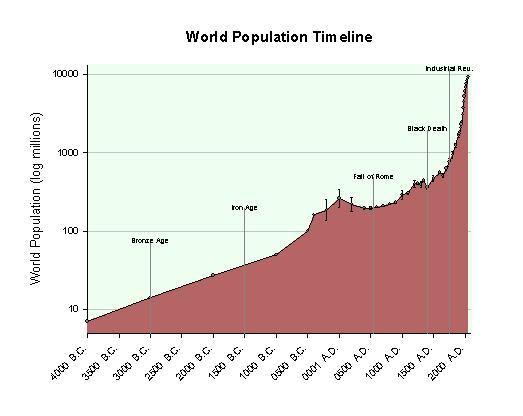Example 9:
World Population Timeline

Plot type: Grouped Error Bars
Data format: X, Many Y Replicates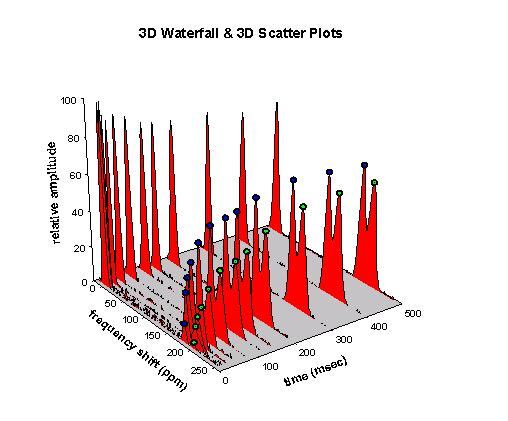Example 10:
3D Waterfall & 3D Scatter Plots

2 Plots in Graph
Plot 1: 3D Waterfall Plot, XY Many Z
Plot 2: 3D Scatter Plot, XYZ Triplet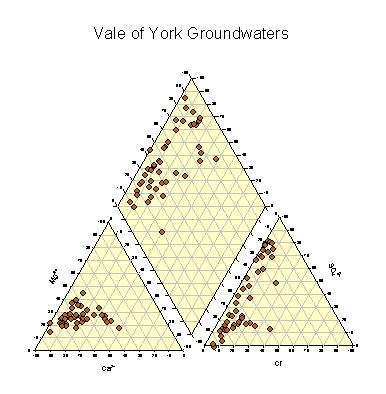Example 11:
Piper Plot

4 Graphs on the Page
Graph 1: Scatter, Ternary Triplet
Graph 2: Scatter, Ternary Triplet
Graph 3: Ternary, XY Pairs
Graph 4: 3 Plots, Simple Scatter, XY Pair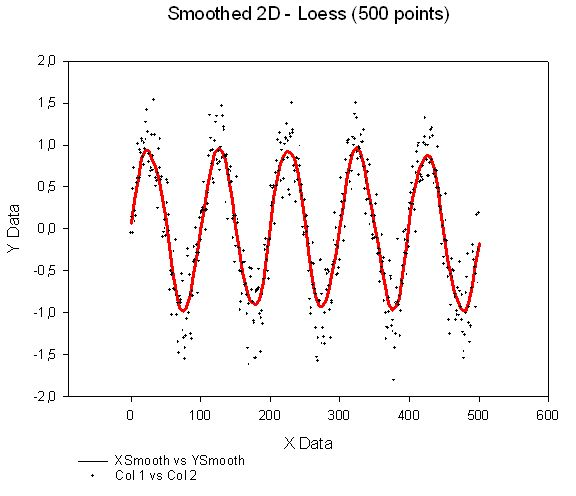Example 12:
2D Smoothed 500 - Loess

Plot 1: Simple Straight Line, XY Pair
Plot 2: Simple Scatter, XY Pair
(-> Analysis > Smoothers)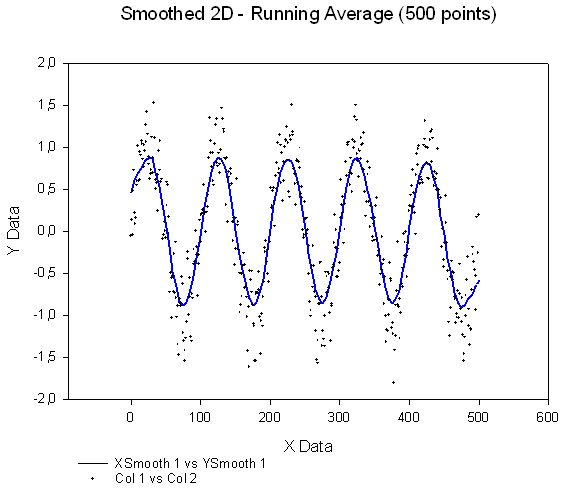Example 12:
2D Smoothed 500 - Running Average

Plot 1: Simple Straight Line, XY Pair
Plot 2: Simple Scatter, XY Pair
(-> Analysis > Smoothers)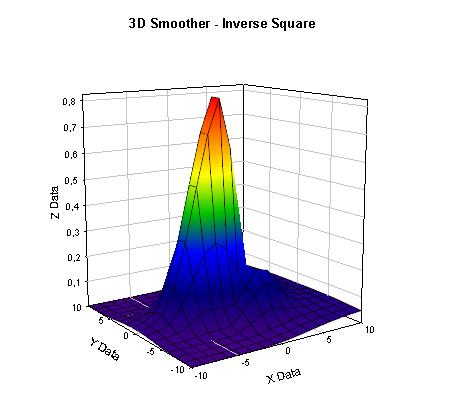Example 13:
3D Smoothed - Inverse Square

Plot 1: Simple Straight Line, XY Pair
Plot 2: Simple Scatter, XY Pair
(-> Analysis > Smoothers)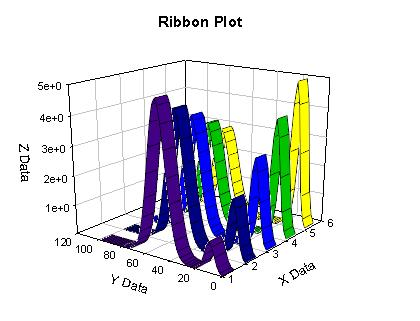Example 14:
Ribbon Plot

Plot 1: 3D Mesh Plot, XYZ Triplet
Plot 2: 3D Mesh Plot, XYZ Triplet
Plot 3: 3D Mesh Plot, XYZ Triplet
Plot 4: 3D Mesh Plot, XYZ Triplet
Plot 5: 3D Mesh Plot, XYZ Triplet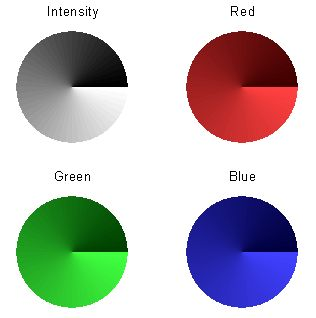Example 15:
Color Wheels

Pie Chart, Single Column
Graph 1: Intensity
Graph 2: Red
Graph 3: Green
Graph 4: Blue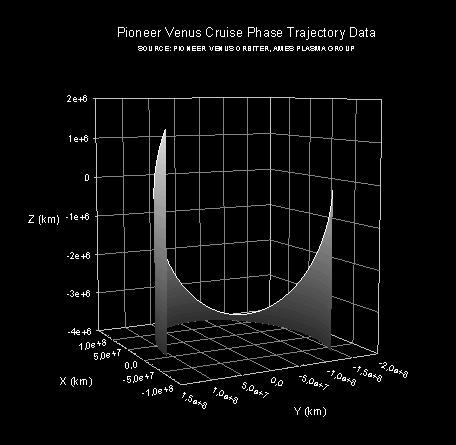Example 16:
Trajectory Plot

Plot type: 3D Trajectory Plot
Data format: XYZ Triplet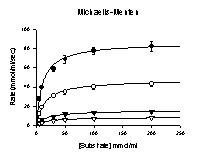Example 17:
Enzyme Kinetics Graphs: Michaelis-Menten

Plot 1-4: Simple Error Bars, X, Many Y Replicates
Plot 5: Multiple Straight Lines, X Many Y
(-> ToolBox > Enzyme Kinetics macro)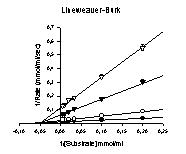Example 17:
Enzyme Kinetics Graphs: Lineweaver-Burk

Plot 1-4: Simple Error Bars, X, Many Y Replicates
Plot 5: Multiple Straight Lines, X Many Y
(-> ToolBox > Enzyme Kinetics macro)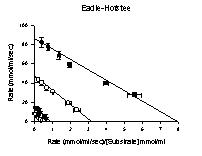Example 17:

Plot 1-4: Simple Error Bars, X, Many Y Replicates
Plot 5: Multiple Straight Lines, X Many Y
(-> ToolBox > Enzyme Kinetics macro)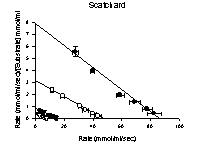Example 17:
Enzyme Kinetics Graphs: Scatchard

Plot 1-4: Simple Error Bars, X, Many Y Replicates
Plot 5: Multiple Straight Lines, X Many Y
(-> ToolBox > Enzyme Kinetics macro)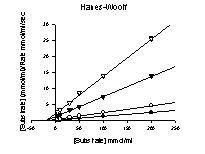Example 17:
Enzyme Kinetics Graphs: Hanes-Woolf

Plot 1-4: Simple Error Bars, X, Many Y Replicates
Plot 5: Multiple Straight Lines, X Many Y
(-> ToolBox > Enzyme Kinetics macro)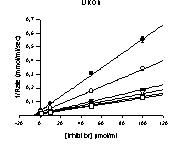Example 17:
Enzyme Kinetics Graphs: Dixon

Plot 1-4: Simple Error Bars, X, Many Y Replicates
Plot 5: Multiple Straight Lines, X Many Y
(-> ToolBox > Enzyme Kinetics macro)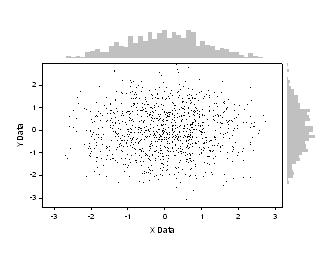Example 18:
Border Plots, Histogram

Graph 1: Simple Scatter, XY Pair
Graph 2: Simple Bar, Single Y
Graph 3: Simple Bar, Single X
(-> ToolBox > Border Plots macro)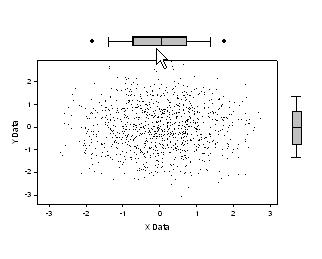Example 18:
Border Plots, BoxPlot

Graph 1: Simple Scatter, XY Pair
Graph 2: Horizontal Box Plot, Many X
Graph 3: Vertical Box Plot, Many Y
(-> ToolBox > Border Plots macro)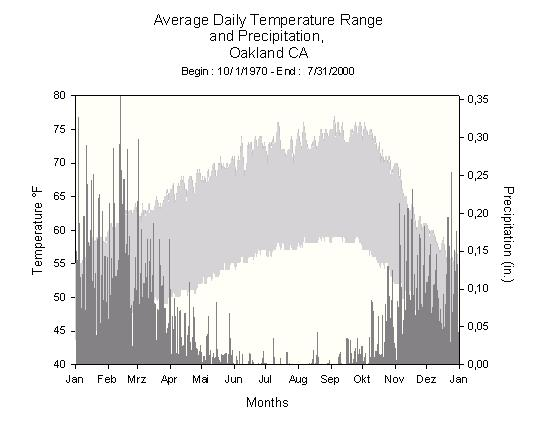Example 19:
Climograph

Plot 1: Multiple Area, X Many Y
Plot 2: Simple Bar, XY Pair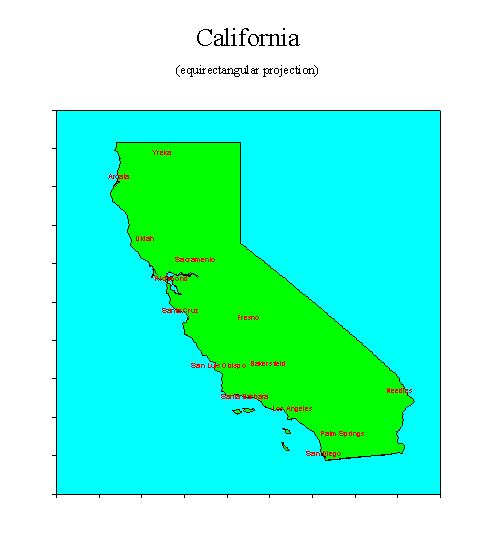Example 20:
California Map

Plot 1, Borders: Vertical Area, YX Pair
Plot 2, Islands: Multiple Area, XY Pairs
Plot 3, Rivers (Hidden): Simple Straight Line, YX Pair
Plot 4, Cities: Simple Scatter, XY Pair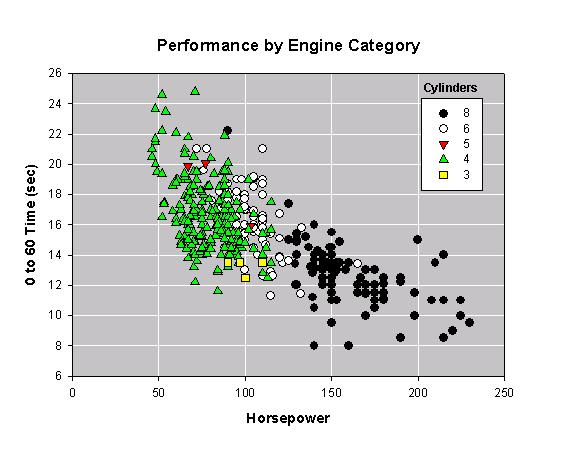Example 21:
Category Graph

Plot type: Multiple Scatter
Data format: XY Category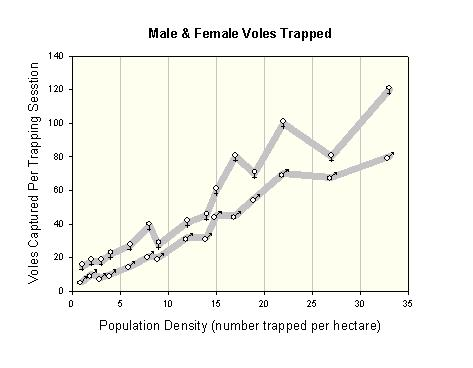Example 22:
Symbols From a Column

Plot type: Multiple Straight Lines
Data format: X Many Y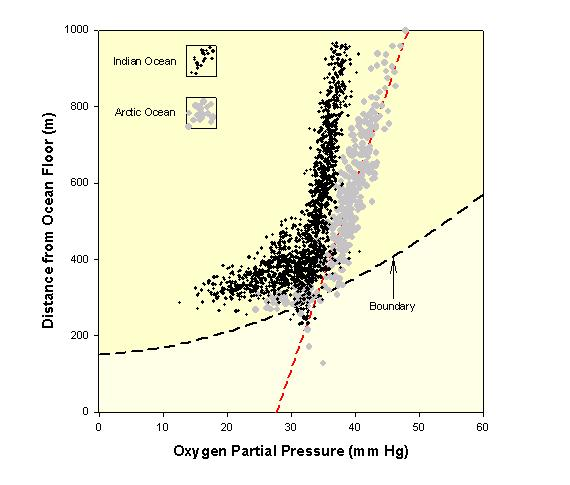Example 23:
Area and Scatter Plots

Plot 1: Simple Straight Lines, XY Pair
Plot 2: Simple Area, XY Pair
Plot 3: Simple Regression, XY Pair
Plot 4: Simple Scatter, XY Pair
Plot 5: Simple Scatter, XY Pair
Plot 6: Simple Scatter, XY Pair
Plot 7: Simple Scatter, XY Pair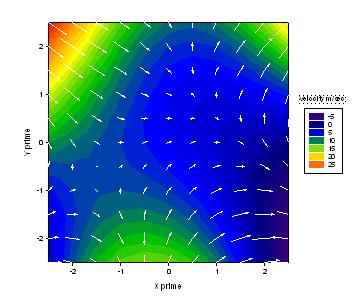Example 24:
Vector Field Plus Contour

Graph 1: Filled Contour Plot, XYZ Triplet
Graph 2: Vector plot XYAM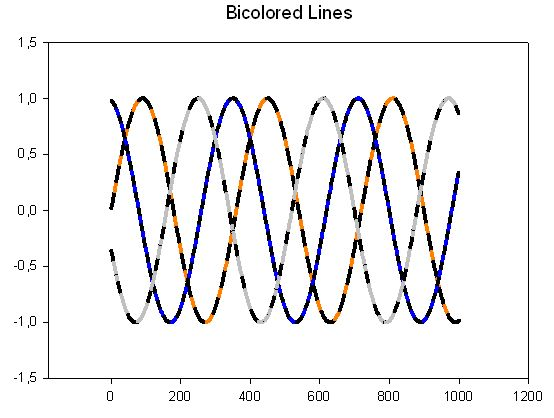Example 25:
Bicolored Lines

Plot 1: Simple Straight Line, XY Pair
Plot 2: Simple Straight Line, XY Pair
Plot 3: Simple Straight Line, XY Pair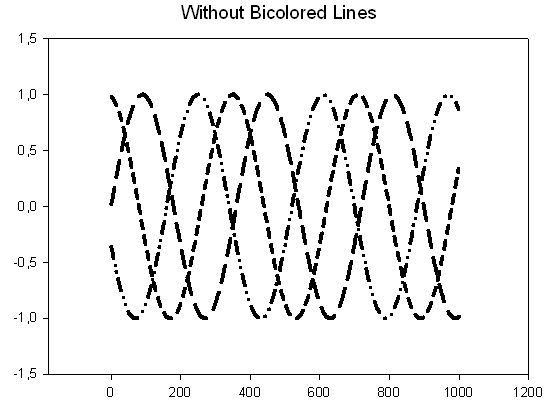Example 25:
Bicolored Lines

Plot 1: Simple Straight Line, XY Pair
Plot 2: Simple Straight Line, XY Pair
Plot 3: Simple Straight Line, XY Pair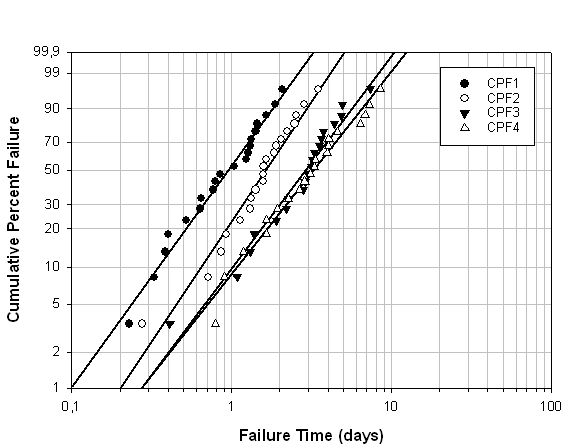Example 26:
Weibull Failure Graph

Plot type: Multiple Regression
Data format: XY Pairs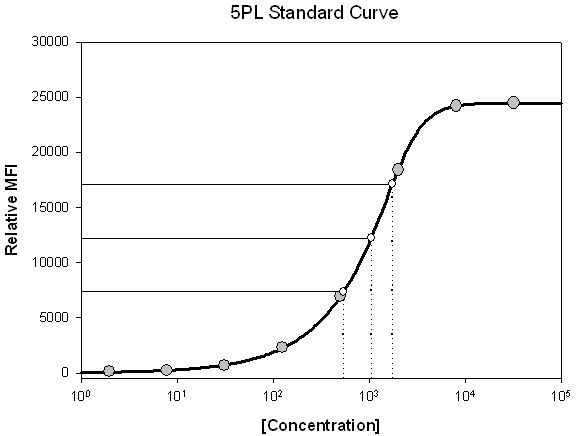Example 27:
5PL Standard Curve

Plot 1: Simple Scatter, XY Pair
Plot 2: Simple Straight Line, XY Pair
Plot 3: Simple Scatter, XY Pair
(-> ToolBox > Standard Curves macro)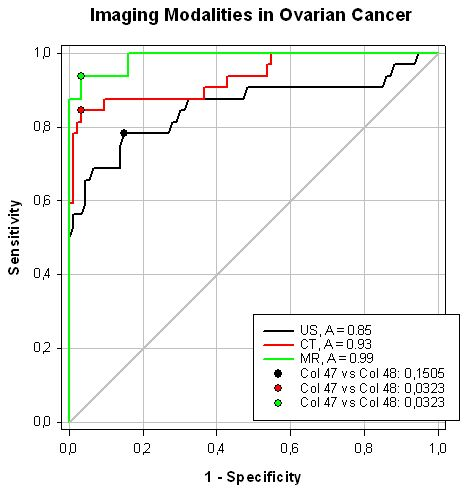Example 28:
ROC Curves

Plot 1: Multiple Straight Lines, XY Pair
Plot 2: Simple Straight Line, XY Pair
Plot 3: Simple Scatter, XY Pair
(-> ToolBox > ROC Curves macro)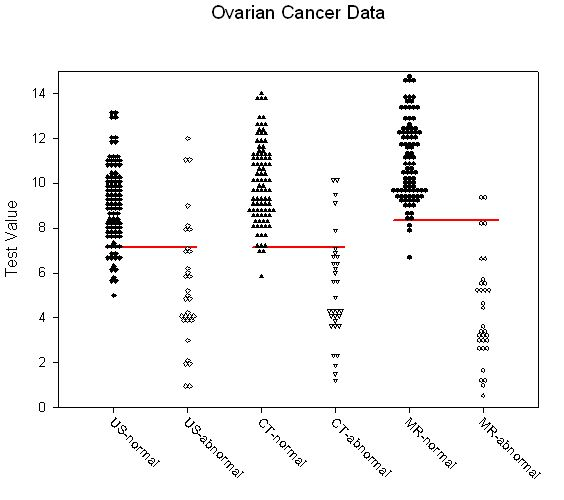Example 28:
ROC Curves, Dot Histogram

Plot 1: Multiple Scatter, XY Pairs
Plot 2: Multiple Straight Lines, XY Pairs
(-> ToolBox > ROC Curves macro)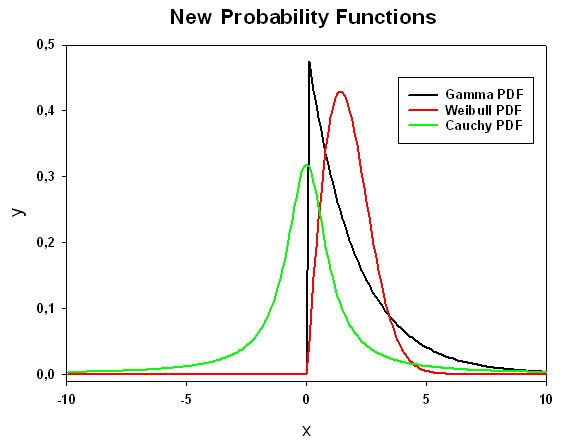Example 29:
Probability Functions: Gamma, Weibull, Cauchy

Plot type: Multiple Straight Lines
Data format: X Many Y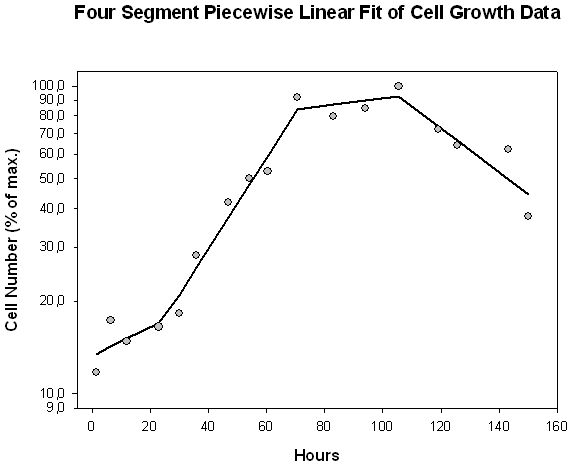Example 30:
Piecewise Linear Fit

Plot 1: Simple Scatter, XY Pair
Plot 1: Simple Straight Line, XY Pair
(-> Analysis > Regression Wizard > Equation Category > Piecewise)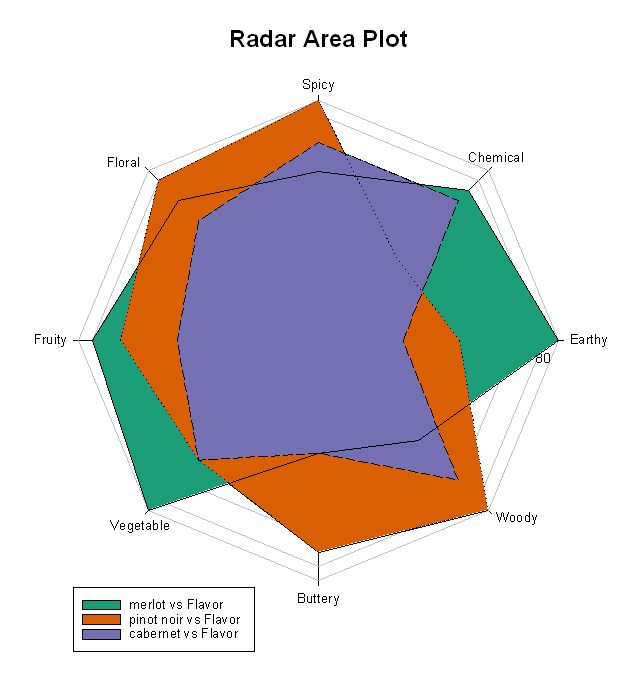Example 31:

Data format: XY Pair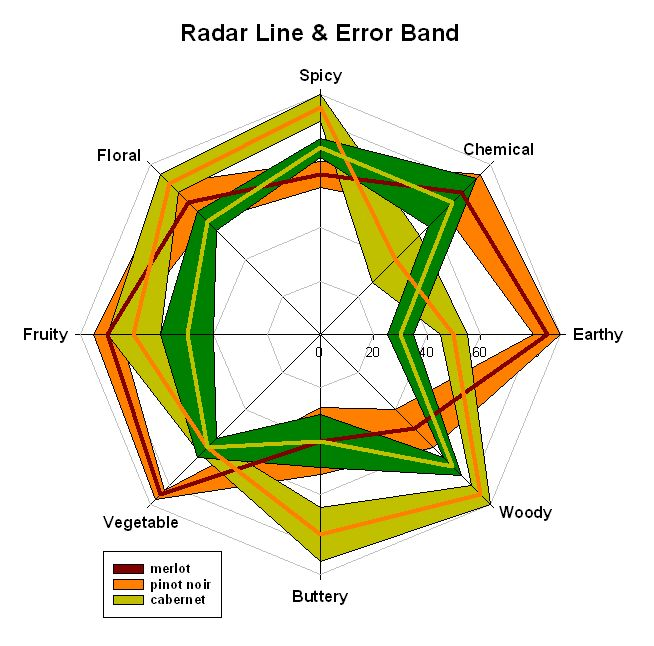Example 31:

Plot type: Radar Line and Error Band
Data format: Label Many Series Error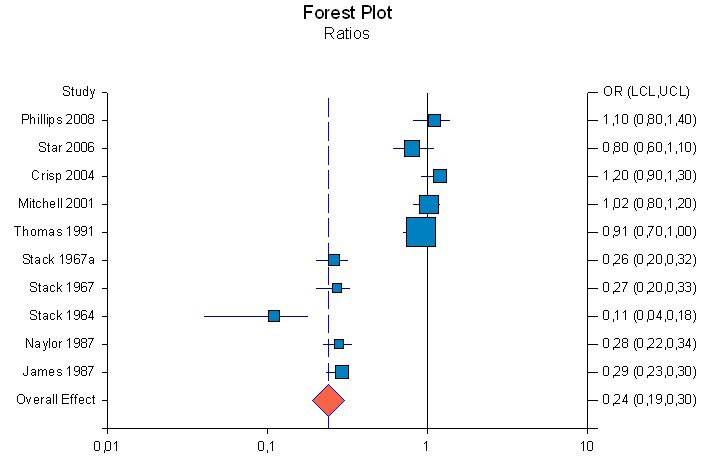Example 32:
Forest Plot Ratios

Plot type: Forest Plot
Data format: Mean difference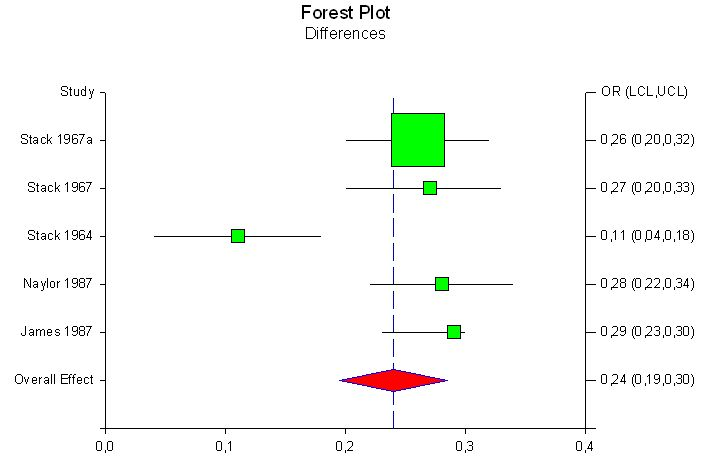Example 32:
Forest Plot Differences

Plot type: Forest Plot
Data format: Mean difference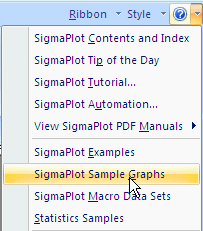Sie können die Datei Samples.jnb vom Help-Menü aus öffnen. Sie liegt im Benutzerverzeichnis: Dokumente\SigmaPlot\SPW14\Samples.

Eine "Masterkopie" finden Sie im Verzeichnis Programme\SigmaPlot\SPW14\Samples.#### SigmaPlot-Produktseite

Tel: +49 (0) 211-5403 9646